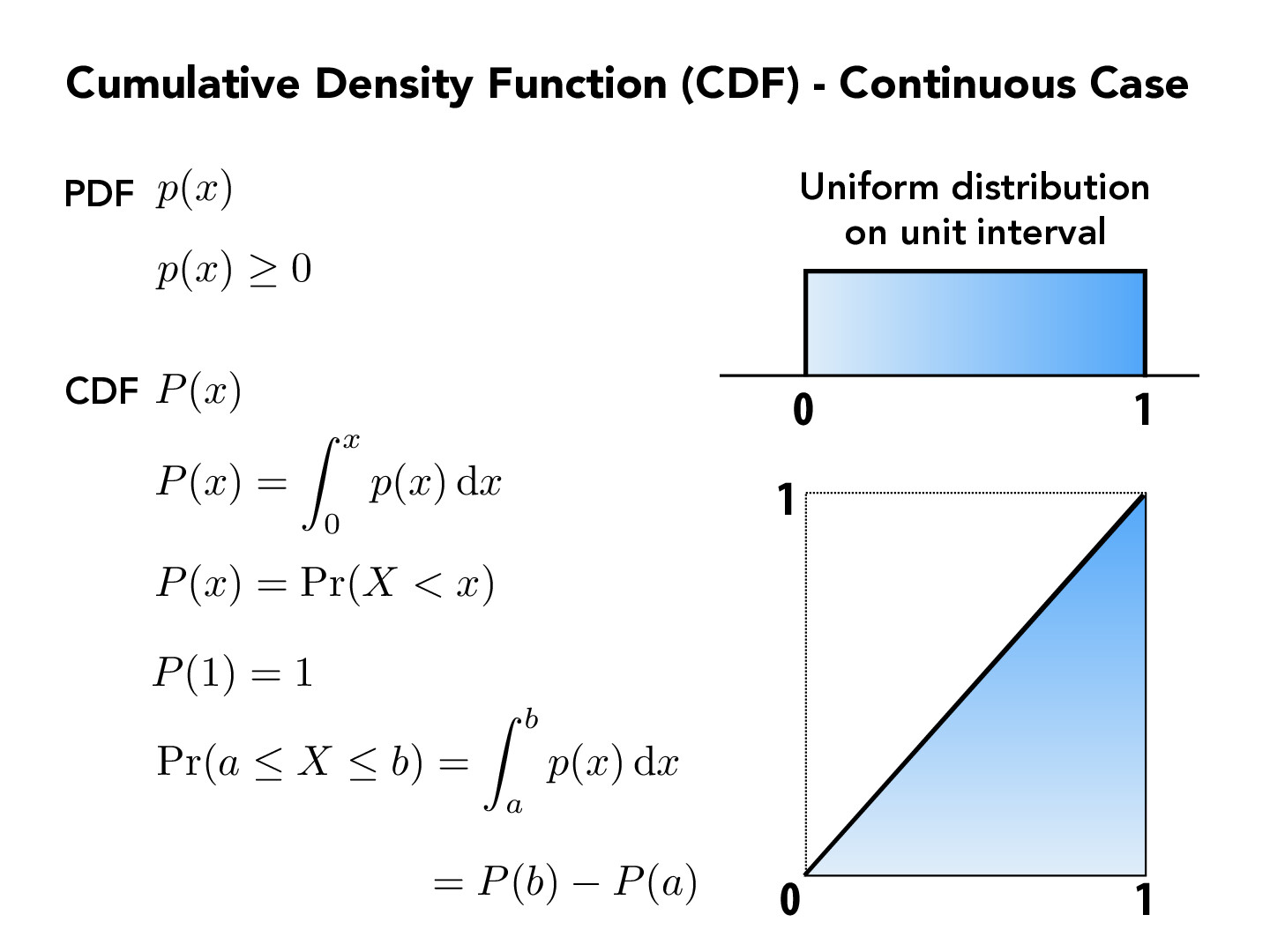Lecture 12: Monte Carlo Integration (51)andrewhuang56

Given our observations in slide 40, 41, and 42, it makes me believe that a $p$ function that resembles the original $f$ function (off by a scale factor) is probably best for estimating the integral of $f.$ Indeed, then, the higher the value of $f$ (so the more impact it has on the integral value), the more we sample at it. However, actually choosing $p(x) = f(x) / c$, where $c$ is a constant, feels so unhelpful/pointless to me: in order to sample in accordance with the $p$ distribution, we'd need to know the CDF of $p$, or $\int_a^r p(x)\, dx$ for different values of $r$. Since $p$ is a scale factor of $f,$ this is essentially the same as just finding the integral of $\int_a^r f(x) \, dx,$ which is what we're setting out to do in the first place (in fact, the value of $c$ that is the scale factor for $p(x)$ is simply $\int_a^b f(x) \, dx$!)

So obviously, we don't want $p(x) = \frac{f(x)}{\int_a^b f(x)\, dx}.$ However, even estimating $p(x)$ as approximately $\frac{f(x)}{\int_a^b f(x)\, dx}$ seems inefficient, as I believe means that we are essentially estimating $f(x)$ and therefore $\int_a^b f(x)$ in the process anyways. So, I guess my question is: in practice, how do we actually get a good enough function for $p(x)$? Clearly, we don't want a very very good function; how do we decide what's good enough with respect to efficiency?

You must be enrolled in the course to comment